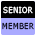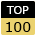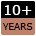Welcome to WebmasterWorld Guest from 54.227.157.163

Forum Moderators: coopster & jatar k

Message Too Old, No Replies

Coding a step function

wheel

8:15 pm on Sep 9, 2008 (gmt 0)

Senior Memberjoined:Feb 11, 2003
posts:5072

I'm trying to write specs to solve an equation with one variable. Problem is, part of the equation is a step function that depends on the value being solved.

I'll use tax rates as an example since it's got kind of a step function in it.

Say we want to have a net income (given). We want to calculate gross.
Net = Gross - Taxes
But taxes are a function of gross income, so it's really
Net=Gross - Taxes(Gross)

Where taxes are a function of gross income.
Lets say something like this:
On first 10,000 of gross, taxes are 0
On next 90000 of gross, taxes are 30%
Anything above 100,000 taxes are 40%.

But I'm not seeing how to calculate taxes(gross) since we don't know gross.

I suppose I could do some sort of iterative targetting to find the answer, but I have this nagging feeling there's a way to do this. Any thoughts?

PHP_Chimp

8:25 pm on Sept 9, 2008 (gmt 0)

Senior Memberjoined:July 12, 2007
posts:766

``function calculateNet(\$gross) {if (\$gross < 10001) {\$net = \$gross;}elseif (\$gross > 10000 && \$gross < 90001) {\$value = \$gross - 10000;\$net = (\$value * 0.3) + 10000;}else {\$value = \$gross - 10000;\$valueNet = (\$value * 0.3) + 10000;\$value1 = \$gross - \$valueNet;\$net = \$value1 * 0.4}return \$net;}``

Or something like that. There is probably a better way to do this, however this is one way.

[edited by: PHP_Chimp at 8:32 pm (utc) on Sep. 9, 2008]

wheel

10:54 pm on Sept 9, 2008 (gmt 0)

Senior Memberjoined:Feb 11, 2003
posts:5072

Thanks :). Except Net is an input - it's a fixed, given number. We need to calculate gross, where gross=net + taxes; taxes being a function of gross. So rearranging:
gross + taxes(gross)=net

where net is known ahead of time, and taxes are a function of gross in that funky step patter I noted above. The basic idea is easy enough, I just expand out the taxes function into an equation. Problem is the taxes function, if I write it out generally, ends up being a bunch of if statements - these statements don't easily reverse engineer into something we can solve.

Does that make any sense at all?

MattAU

3:54 am on Sept 10, 2008 (gmt 0)

Junior Memberjoined:June 6, 2005
posts:109

This is actually a simple problem, yet it can be very difficult to work out if you try and think about it too hard... :)

When you multiple by something you divide by the same amount to get back to where you started, and vice versa.

90000 * 0.7 = 63000
63000 / 0.7 = 90000

So you take you net value... Say 80000:

\$net = 80000;
\$gross = 0;

> Bracket 1
\$gross = 0 + 10000 = 10000
\$net = 80000 - 10000 = 70000

> Bracket 2
\$gross = 10000 + (63000 / .7) = 100000;
\$net = 70000 - 63000 = 7000;

> Bracket 3
\$gross = 100000 + (7000 / .6) = 111667;
\$net = 7000 - 7000 = 0;

> Gross = 111667

:)

wheel

2:17 pm on Sept 10, 2008 (gmt 0)